# JEE Mathematics Definite Integral MCQs Set B

JEE Mathematics Definite Integral MCQs Set B with answers available in Pdf for free download. The MCQ Questions for JEE Mathematics with answers have been prepared as per the latest JEE Mathematics Definite Integral syllabus, books and examination pattern. Multiple Choice Questions form important part of competitive exams and JEE exam and if practiced properly can help you to get higher rank. Refer to more topic wise JEE Integrals Questions and also download more latest study material for all subjects and do free JEE Mathematics Mock Test

## Definite Integral JEE Mathematics MCQ

JEE Mathematics Integrals students should refer to the following multiple-choice questions with answers for Definite Integral in JEE. These MCQ questions with answers for JEE Integrals will come in exams and help you to score good marks

### Definite Integral MCQ Questions with Answers

#### Question: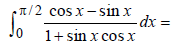• a) 0
• b) 2
• c) – 2
• d) None of these

#### Question: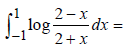• a) 0
• b) 1
• c) 2
• d) – 1

#### Question: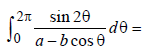• a) 0
• b) 2
• c) 1
• d) None of these

#### Question: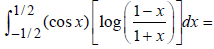• a) 0
• b) 1
• c) e1/2
• d) 2e1/2

#### Question: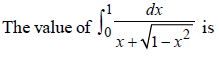• a)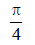• b)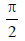• c)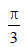• d) 1/2#### Question: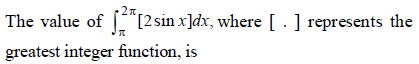• a)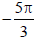• b)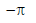• c)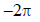• d)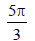#### Question: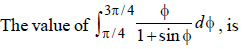• a)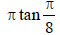• b)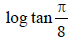• c)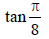• d) None of these#### Question: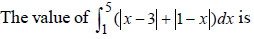• a) 12
• b) 10
• c) 21
• d) 5/6

#### Question: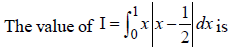• a) 1/8
• b) 1/3
• c) 1/4
• d) None of these

#### Question: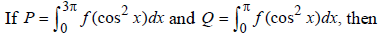• a) P - 3Q = 0
• b) P -Q = 0
• c) P - 2Q = 0
• d) P - 5Q = 0

Answer: P - 3Q = 0

## More Questions..............................

#### Question: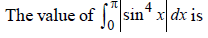• a)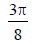• b)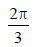• c)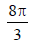• d) None of these#### Question: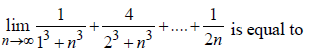• a)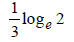• b)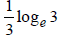• c)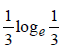• d) None of these#### Question: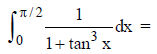• a)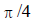• b)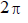• c) 0
• d) 1#### Question: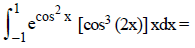• a) 0
• b) 1
• c)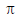• d)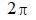#### Question: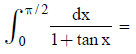• a)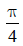• b)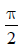• c)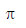• d)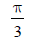#### Question: For any integer n,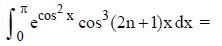• a) 0
• b) 1
• c) none of these
• d)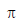#### Question: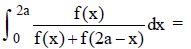• a) a
• b) 0
• c) 2a
• d) none of these

#### Question: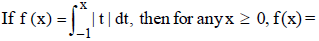• a)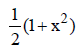• b)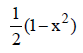• c) 1– x2
• d) 1 + x2#### Question: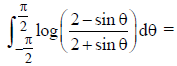• a) 0
• b) 2
• c) 1
• d) none of these

#### Question: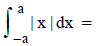• a) a2
• b) 0
• c) a2/2
• d) a2/4

#### Question: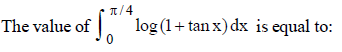• a)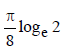• b)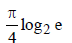• c)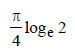• d)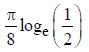#### Question: Let f (x) be a nonconstant twice derivable function defined on R such that f (2 + x) = f (2 – x) and f ' (1/2) = 0 = f ' (1). Then which of the following alternative(s) is/are correct ?

(1) f (– 4) = f (8)
(2) Minimum number of roots of the equation f '' (x) = 0 in (0, 4) are 4.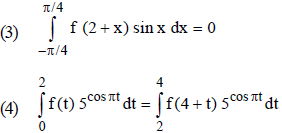• a) 1, 2 and 3 are correct
• b) 2 and 4 are correct
• c) 1 and 2 are correct
• d) 1 and 3 are correct

Answer: 1, 2 and 3 are correct

#### Question: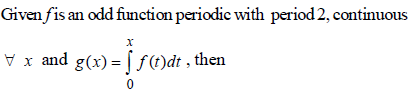(1) g (2n) = 0                 (2) g(x) is even function
(3) g (x) is odd function (4) g (2n) = 1

• a) 1 and 2 are correct
• b) 1 and 3 are correct
• c) 1, 2 and 3 are correct
• d) 2 and 4 are correct

Answer: 1 and 2 are correct

#### Question: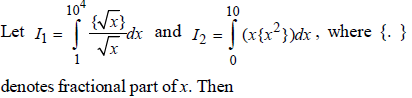(1) I1 = I2  (2) I1 = 4I2
(3) I1 < I2 (4) I1 = 100

• a) 2 and 4 are correct
• b) 1, 2 and 3 are correct
• c) 1 and 2 are correct
• d) 1 and 3 are correct

Answer: 2 and 4 are correct

#### Question: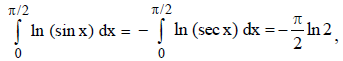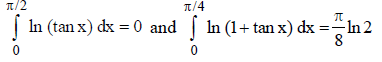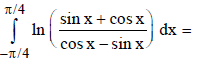• a) 0
• b)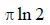• c)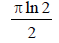• d)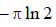#### Question: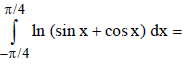• a)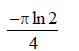• b)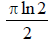• c)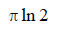• d) 0#### Question: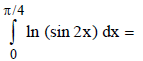• a)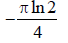• b)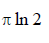• c)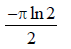• d)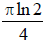#### Question: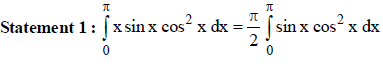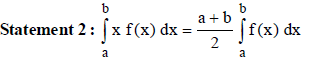• a) Statement -1 is True, Statement-2 is False.
• b) Statement -1 is False, Statement-2 is True.
• c) Statement-1 is True, Statement-2 is True; Statement-2 is NOT a correct explanation for Statement-1.
• d) Statement-1 is True, Statement-2 is True; Statement-2 is a correct explanation for Statement-1.

Answer: Statement -1 is True, Statement-2 is False.

#### Question: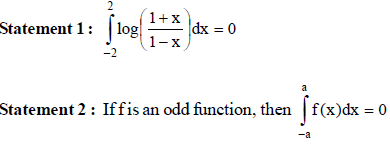• a) Statement-1 is True, Statement-2 is True; Statement-2 is a correct explanation for Statement-1.
• b) Statement-1 is True, Statement-2 is True; Statement-2 is NOT a correct explanation for Statement-1.
• c) Statement -1 is False, Statement-2 is True.
• d) Statement -1 is True, Statement-2 is False.

Answer: Statement-1 is True, Statement-2 is True; Statement-2 is a correct explanation for Statement-1.

#### Question: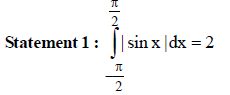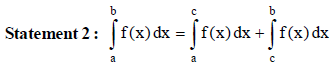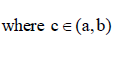• a) Statement-1 is True, Statement-2 is True; Statement-2 is a correct explanation for Statement-1.
• b) Statement-1 is True, Statement-2 is True; Statement-2 is NOT a correct explanation for Statement-1.
• c) Statement -1 is False, Statement-2 is True.
• d) Statement -1 is True, Statement-2 is False.

Answer: Statement-1 is True, Statement-2 is True; Statement-2 is a correct explanation for Statement-1.

## Tags:

Click for more Integrals Study Material
 JEE Mathematics Definite Integral MCQs Set B JEE Mathematics Indefinite Integrals MCQs Set A JEE Mathematics Application of Integrals MCQs JEE Mathematics Indefinite Integrals MCQs Set B JEE Mathematics Indefinite Integrals MCQs Set C JEE Mathematics Indefinite Integral MCQs Set A JEE Mathematics Indefinite Integral MCQs Set B JEE Mathematics Definite Integration MCQs Set A JEE Mathematics Definite Integral MCQs Set A JEE Mathematics Definite Integration MCQs Set B

## Latest NCERT & CBSE News

Read the latest news and announcements from NCERT and CBSE below. Important updates relating to your studies which will help you to keep yourself updated with latest happenings in school level education. Keep yourself updated with all latest news and also read articles from teachers which will help you to improve your studies, increase motivation level and promote faster learning

### MCQ Question based CBSE examination

For 2021-22 CBSE has launched MCQ question-based examination for Term 1 & Term 2 board examinations. The entire syllabus has been divided into two parts each including 50% of the entire syllabus. To score well, students must practice as per the new CBSE term-wise...

### Score well in Class 12 English Boards Exam

12th Board exams are an important part of students' lives. The marks obtained in the board exam decide the college in which one can study. In class 12 the syllabus of each and every subject increases vastly and it is difficult to cover up every point. In English also...

### How To Solve Unseen Passages In English

Unseen passages may contain one or many paragraphs. This is one of the important yet easy parts for a student to get marks. Students should thoroughly study and understand the passage to answer the related questions. The unseen passages are there just to test the...

### CBSE Class 10 Revised Syllabus

Last year CBSE had to reduce the syllabus because of the pandemic situation but it was not very effective because there were no examinations. This year to avoid any confusion and conflict, CBSE has decided to reduce the syllabus into term 1 and term 2. 50 percent of...

×